#### Visual Basic Programming

This tutorial is ideal for:

• Those who learn Visual Basic as a part of GCSE Computer Science course- OCR, AQA and Edexcel
• Those who learn Visual Basic as a part of AS/A Level Computer Science course - OCR, AQA and Edexcel
• A complete novice who wants to learn the nitty-gritty of computer programming
• Those who want a springboard for OOP - Object Oriented Programming
• Those who want to learn Visual Basic quickly on this page - interactively on a real VB Console

Visual Basic, widely known as Visual Basic.net or VB.Net, is a very simple programming language to learn, mimic real-life situations and develop high level applications in Microsoft's .Net platform; it is a popular language, as it is closer to plain English and resembles pseudo code.

If you have a passion for programming, this tutorial will give you much needed impetus for reaching the ultimate goal, even if you are a complete beginner. If you are a seasoned programmer from another background, this is ideal for a new start.

First of all, here is the link to download a less complex Visual Studio for a Windows PC.

Once installed, start Visual Studio and you will see the following screen.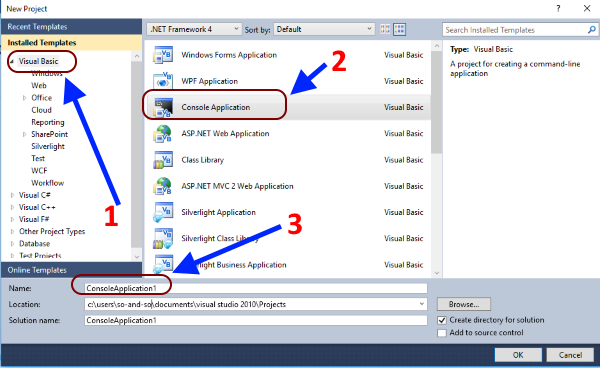Choose a console application and you are ready to experience a great learning learning curve.

Alternatively, you can use the following online console for practising interactively here.

Most of this tutorial is going to be in the interactive mode - the easier phase.

#### Programming in Interactive Mode with the online console

In interactive mode, console can print a line as follows:

Sub Main()
document.WriteLine("This is my first line of code.")
End Sub

Make sure the code is written between Sub Main() ... End Sub for it to work.

The first line of code writes the statement on the screen; the second line reads it and make it visible on the screen. Without the second line, there will be a short flash, indicating the execution of the line, but will not to be visible to the user. So, it's important to carry at least one second line on the screen to see the outcome of our lines of code.#### Commenting things out...

More often than not, we have to comment things out in our code for the following reasons:

• To remind us at a later stage of what the code lines are supposed to do.
• To make the a member of a team aware of what a section of the code actually does in the programme.
• Make the readability of the code more efficient.

This is all done by the humble, old apostrophe - ' in Visual Basic. The following image shows the role of a comment.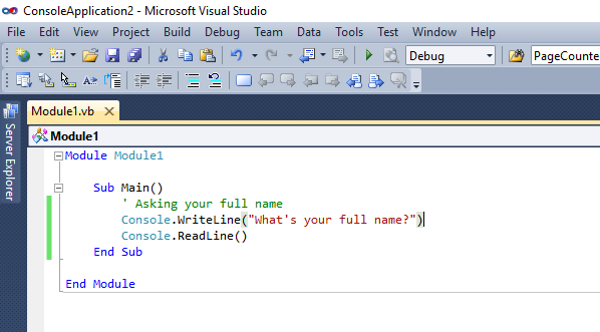The comments are not executed and will not affect the flow of the programme.

#### Storing the Input...

Look at the following piece of code in action:

Sub Main()
End Sub

In the above example, a user enters first name and surname in the hope of seeing his full name being printed. This is what you actually happens: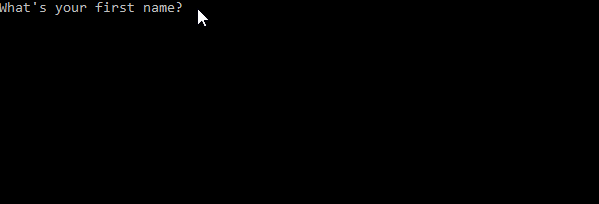As you can see, the full name is not printed despite the first name and surname being given. Why?

Because, the computer hasn't stored the first name and last name in its memory, to be recalled for printing. In short, when it comes to the fifth line, it doesn' know what to print. In order to address this issue, we have to learn about variables.

#### Variables - the containers in memory!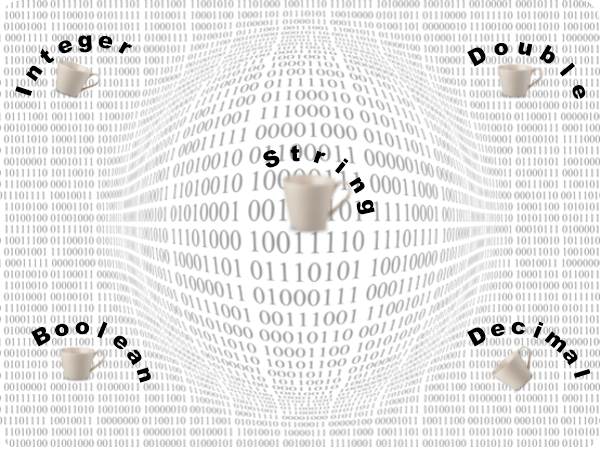Variables store the information or data in the memory and act as containers. The type of the container determines what it can store. These are some of the types of variables in programming languages:

• String - e.g. "Hello!", for string variables
• Double - e.g. 3.1427, for floating point numbers
• Integer - e.g. 3, for integers
• Boolean - True or False

In real life, if a sieve is a container, it cannot hold water, but it can hold ice. Exactly in the same way, an integer type cannot hold a string or vice versa. In short, the variable type does matter, when it comes to storing data.
In addition, when you can carry something in a carrier bag, you don't use a sack for the same purpose. In this context, the choice of the right variable - even in numbers like 4, 50000 or 3.786 - determines how efficiently a programme uses memory resources.

#### Use of Variables

Strings

Let's use the variables, string, to store the first name, the last name and then print the full name. Please note the way a space is inserted by the use of " ", between the two variables.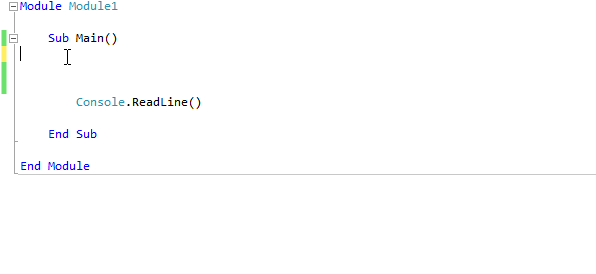In the above example, the first name and last name are stored in two string variables, to be printed later as the full name.

Integers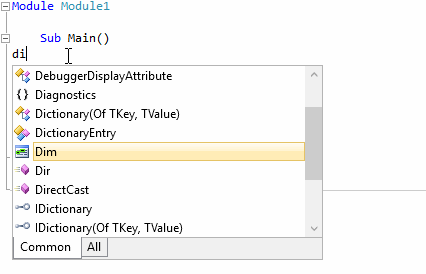In the above example, two integers are stored in the variables, a and b, then added them up and the sum is stored in the variable, c, to be printed.

DoubleThe role of the variable, double, is explained in the above example, by printing the value of π.

Boolean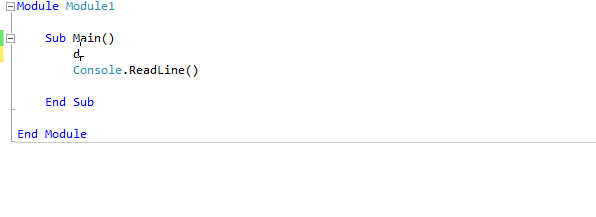Boolean variables store the values as, True or False.

#### Parameters for Functions and Sub Procedures - By Val and By Ref

Making a distinction between parameter paradigm, By Val and By Ref, is something that even seasoned programmers find difficult to explain. The following animation will make it explicitly clear:ByVal is the type of a parameter by default; it means a copy of the actual variable is passed as the parameter to a function or sub procedure, while leaving the original variable unchanged.

ByRef, on the other hand, hands over the memory location of the variable as the parameter, rather than a copy of the variable to a function or a sub procedure. That means the variable changes after the execution of the function or sub procedure.

In the above example, SwapNumbers() sub procedure shows the difference: When parameters are passed by ByVal, they are not swapped around.
When parameters are passed by ByRef, they are swapped around.#### String Concatenation

In Visual Basic - or any other programming language - there are plenty of ways to 'manipulate' strings, known as concatenation. It is extremely vital, regardless of the programming language.

In the following image, you can get an idea about a few ways of manipulating the same string, which could be a springboard to master concatenation.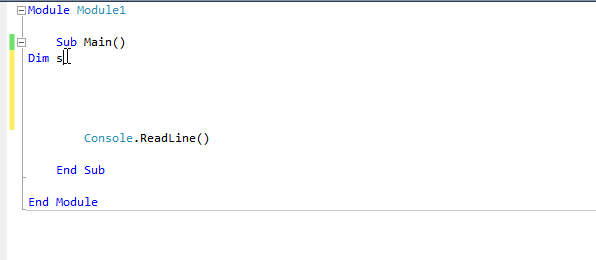As you can see, all three strings produce the same outcome. This is string concatenation.

E.g.
The following approach is always useful in successfully concatenating strings: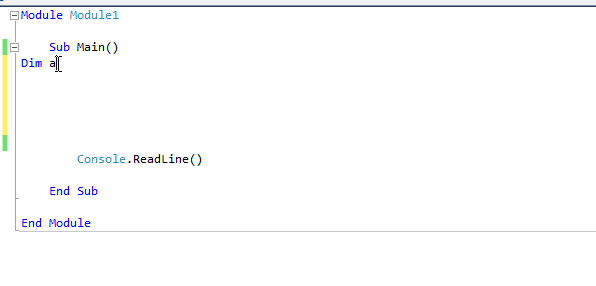#### String Formatting

The following code shows how to format a string for the output using curly brackets, {}, inside a string:

Sub Main()

Dim FirstName as String
Dim LastName as String
FirstName="Tom "
LastName="Cruise"
Console.WriteLine("The Name of the Actor: {0}{1}", FirstName, LastName)

End Sub

Output:
The Name of the Actor: Tom Cruise

The elements, 0 and 1, are provided inside the string as shown above. This approach can be extended to integers - or any other data type - as well well text-muted, which is as follows:

Sub Main()

Dim a as Integer
Dim b as Integer
dim c as Integer a=7
b=5
c=a*b
Console.WriteLine("The product of {0} and {1} = {2}", a, b, c )

End Sub

Output:
The product of 7 and 5 = 35

#### Sub Procedures

A series of instructions that performs a task without returning a values is called a Sub Procedure in Visual Basic. The set of instructions are enclosed between, Sub Procedure-name()...End Sub statements.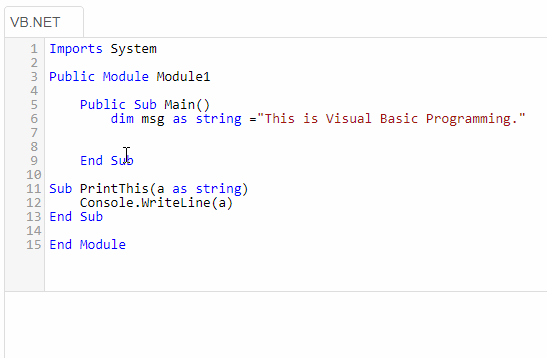In the above animation, the sub procedure, PrintThis(), prints out a message given to it as a parameter; it doesn't return a value, but just prints a statement.

Public Module Module1
Public Sub Main()
dim msg as string ="This is Visual Basic Programming."
PrintThis(msg)
End Sub

Sub PrintThis(a as string)
Console.WriteLine(a)
End Sub
End Module

#### Functions

A function gets an input from a user, then executes a set of lines of code and returns a value for the user to use.
E.g.
When you use the square root function in a calculator, the input is the number that you key in. In a function, this is called, parameter.
The output that you see is called, the return value.

As you know very well well text-muted, if you key in a letter to find a square root, it gives an error. That means the parameter and the return type must match. Please look at the following example:

Function Add_Two_Numbers(a As Integer, b As Integer)
Return a+b
End Function

a and b are parameters - integer type variables, in this case. Return type is an integer too. Let's see the code in action: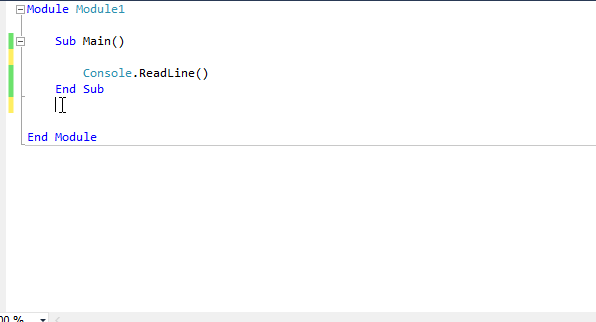Please note that a function must be declared outside Sub Main.....End Sub loop in the console and has to be called in the Sub Main.....End Sub section.

#### Practise the following functions:

You can practise the following functions in the above console or in Visual Studio:

Function Volume_of_Cylinder(radius As Double, height As Double)
End Function

Function Mean_of_Three_Numbers(a As Integer, b As Integer, c As Integer)
Return (a+b+c)/3
End Function

Function Body_Mass_Index(weight_kg As Double, height_m As Double)
Dim c As Double 'declaring a third variable
c=weight_kg/(height_m*height_m)
Return (Int(c*100)/100) ' approximating the return value to one decimal place
End Function

#### Decision Making - If...End If

Decision making is an important element in computer programming. This is how Bill Gates described its significance:

E.g.1 A function to check whether the input is bigger or smaller than 5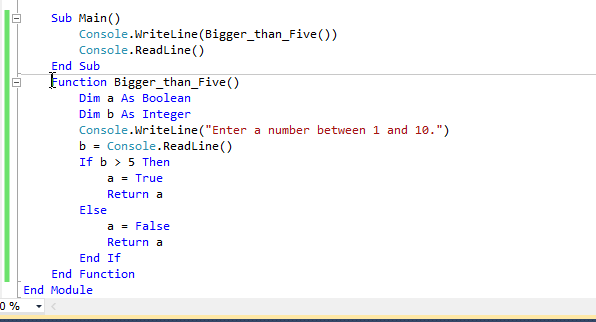Note that the above function returns a Boolean value as the output.

E.g.2 An extended If..End If loop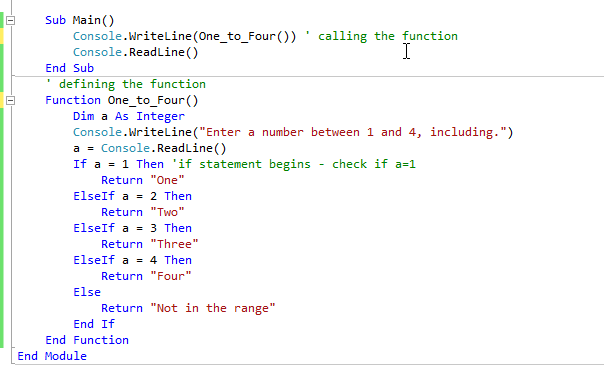E.g.3 Odd Even Checker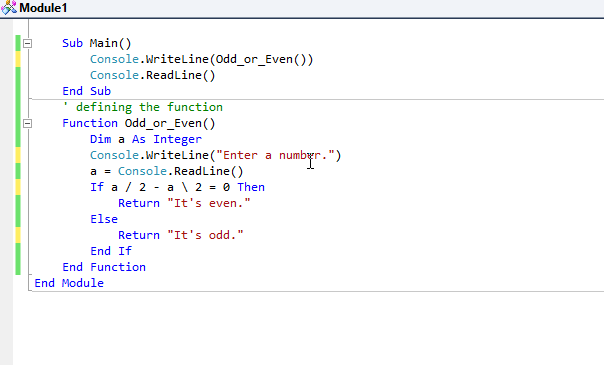#### Loops

Loops form a really important element in computer programming. They let us iterate a given task multiple times, in certain conditions.

E.g. If Mark Zuckerberg, the founder of Facebook, wants to inform his followers a new addition, he can't afford to type in the same thing millions of times. Instead, he just have to write it once, and then make use of a loop to do the rest - in a matter of minutes.

In Visual Basic, we mainly focus on two loops:

• For Loop
• While Loop

For Loop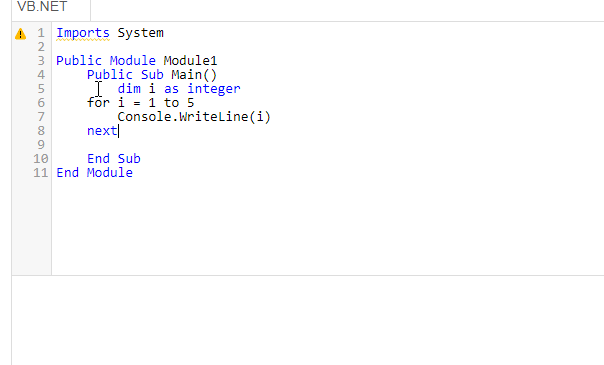You may have seen that step could be used to print the number from 1 to 5 in descending order; it is optional, if i goes up in 1. The animation makes clear the three possibilities.

For Loop - early exist with Exit ... For loopExit For gives an opportunity for us to get out of a loop at a desired point.

Do While Loop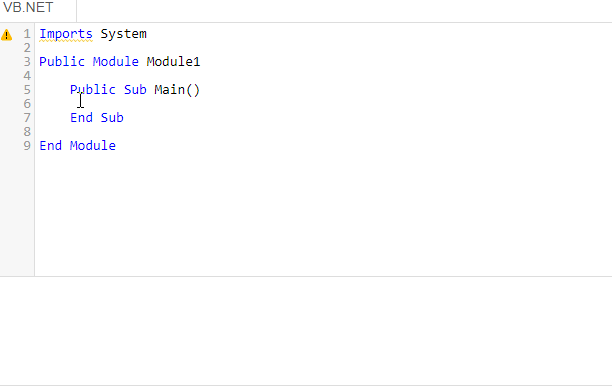The code inside the while loop runs as long as the i value, that goes up by 1 in each iteration, remains less than the value given in the condition.

Do Until LoopThe code inside the while until the i value, that goes up by 1 in each iteration, reaches the condition.

#### Generating Random Numbers

Random number are essential in simulating real-life situations in models. In Visual Basic, Randomize() function, along with Rnd() do just that. The pair generate a number between 0 and 1.

Sub Main()

Dim b As Double
Randomize()
b = Rnd()
Console.WriteLine(b)
End Sub

End Sub

Output:
0.3245 7690 8765 1214

A random number in this form is of little use. So, we can manipulate the above functions to generate random integers which serve bigger purposes, especially in modelling.

E.g. Generating 5 random integers between 0 and 10

Sub Main()

Dim a, i As Integer
For i = 1 To 5
Randomize()
a = Rnd() * 10
Console.WriteLine(a)
Next

End Sub

Output:
3
5
0
7
9

You may have noticed that numbers range from 0 to 10, not including 10. If you want the numbers between 1 and 10, the programme must be as follows:

Sub Main()

Dim a, i As Integer
For i = 1 To 5
Randomize()
a = Rnd() * 10 + 1
Console.WriteLine(a)
Next

End Sub

Output:
8
5
10
7
2

#### Arrays

An array is a list of items: they can be numbers, characters or combinations of both. Each part of an array is called, an element. The first element is known as the 0th element. The number of elements in an array is called, the dimension or the size of the array.

The following code sample,
Declares an integer array of size 5
Stores 5 numbers
And then print them out. Please note the significance of the zero element.

Sub Main()

Dim i As Integer
' An array of integers declared for five elements
Dim number(5) As Integer
' The array is filled with integers
number(0) = 5
number(1) = 10
number(2) = 15
number(3) = 20
number(4) = 25
'Array is printed with the five elements
For i = 0 To 4
Console.WriteLine(number(i))
Next

End Sub

Output:
5
10
15
20
25

The following array is a string array; it stores five car models:

Sub Main()

Dim i As Integer
' An array of strings declared for five elements
Dim car(5) As String
' The array is filled with cars
car(0) = "Ford"
car(1) = "Toyota"
car(2) = "Nissan"
car(3) = "Renault"
car(4) = "Vauxhall"
'Array is printed with the five elements
For i = 0 To 4
Console.WriteLine(car(i))
Next

End Sub

Output:
Ford
Toyota
Nissan
Renault
Vauxhall

Note: array(element number) is the way to refer to an element in Visual Basic, which is different from other programming languages, like Python or JavaScript.

#### Reading an Array with For Each ... Next

This can be used to execute some code while iterating through each element of an array. The following examples illustrate it:

E.g.1 An array of integers

Sub Main()

' An array of strings declared for five elements
Dim primes(5) As Integer
' The array is filled with prime numbers
primes(0) = 2
primes(1) = 5
primes(2) = 7
primes(3) = 11
primes(4) = 17
'Array is printed with the five elements
For each prime in primes
Console.WriteLine(prime)
Next

End Sub

Output:
2
5
7
11
17

E.g.2 An array of strings

Sub Main()

' An array of strings declared for five elements
Dim mammals(5) As String
' The array is filled with mammals
mammals(0) = "Cow"
mammals(1) = "Donkey"
mammals(2) = "Lion"
mammals(3) = "Zebra"
mammals(4) = "Bat"
'Array is printed with the five elements
For each mammal in mammals
Console.WriteLine(mammal)
Next

End Sub

Output:
Cow
Donkey
Lion
Zebra
Bat

#### 2-D Arrays

2-D arrays are useful in storing data in pairs, such as coordinates.

Sub Main()

' Declaring the 2-D array
Dim a(3, 3) As Integer
Dim b As Integer
' Filling up the array
Dim i, j As Integer
For i = 0 To 2
For j = 0 To 2
Randomize()
b = Int(Rnd() * 100)
a(i, j) = b
Next
Next
' Printing the elements of the array
For i = 0 To 2
For j = 0 To 2
Console.WriteLine(a(i, j))
Next
Next

End Sub

Output:
12
45
23
67
89
65
33
78
23

The grid of elements:
(0,0) (0,1) (0,2)
(1,0) (1,1) (1,2)
(2,0) (2,1) (2,2)

The grid of data:
12, 45, 23
67, 89, 65
33, 78, 23

This is 3 X 3 2-D array. It has nine elements to store the random numbers. In order to store the numbers - and recall for printing - each element must be referred to by two numbers, to represent the row value and column value - in a 3 X 3 grid. You may have seen that two nested loops have been used to store and recall data.

#### Structures

In Visual Basic, Structures are called UDT - User Defined Types. They are immensely useful to store a mixture of data types as a single unit.

For instance, suppose a boxing club wants to set up a new database while storing the details of its members, before going online. It can declare a Structure type for the purpose and store the information in it - and use it - as follows:As you can see, so much information can be stored in one variable with this approach.

#### ASCII Code Manipulation with Visual Basic

ASCII code, known as the American Standard Code for Information Interchange, plays a great role in coding. The letters of your keyboard, for instance, have an equivalent number in ASCII code. The ASCII code for the letters, A to Z, range from 65 to 91. When you press, say, A, on the keyboard, a series of binary digits - 1000001 - are generated as electric pulses that go through the circuit of the keyboard to the processor.

In Visual Basic, chr(ascii-number) shows the letter on the screen. On the other hand, Asc("letter") shows the ASCII code.

E.g.
Console.WriteLine(chr(65)) - prints A
Console.WriteLine(chr(97)) - prints a
Console.WriteLine(Asc("A")) - prints 65
Console.WriteLine(Asc("a")) - prints 97

The following code shows the entire alphabet in the uppercase and lowercase:

Sub Main()
For i = 0 To 25
Console.WriteLine(Chr(65 + i) + " , " + Chr(97 + i))
Next
End Sub

Output:
A , a
B , b
C , c
......
X , x
Y , y
Z , z

#### The Caesar Cipher

The Caesar Cipher is one of the oldest cipher algorithms, if not the oldest, known to us. It was the way Julius Caesar, the Roman Emperor, used to communicate with his subordinates while keeping the secrecy intact, over 2000 years ago. This is a simple, fairly straight-forward cipher; therefore, it never lost its potential to inspire millions of programmers across the world in the digital age.

According to the Caesar Cipher, each letter of a sentence is shifted by a certain number, known as the key, to the left or right; this is known as encrypting. If the key is known to the recipient, the change can be reversed and the message can be read easily; this is called, decrypting.

Caesar's Actual Message:FightToTheEnd

ShiftEncrypted Message
1GjhiuUpUifFoe
2HkijvVqVjgGpf
-1EhfgsSnSgdDmc
-3CfdeqQlQebBka

The following function can be used to encrypt a string using the Caesar Cipher. Please note that it's very simplistic - for the audience intended. It just encrypts a simple string that contains the letter of the alphabet and take two parameters - the string to be encrypted and the shift as an integer. Please make sure the sting only contains letters in the lowercase.

Module Module1
Sub Main()
Console.WriteLine(Caesar_Cipher("Hello", 2))
End Sub
Function Caesar_Cipher(str As String, shift As Integer)
Dim cipher As String = ""
Dim chrvalue As Integer
For Each ch In str
chrvalue = Asc(ch) + shift
If chrvalue >= 65 And chrvalue <= 122 Then
cipher += Chr(chrvalue)
Else
cipher = ""
Exit For
End If
Next
Return cipher
End Function
End Module

Output:
Jgnnq

#### Useful Programmes

E.g.1 Times Tables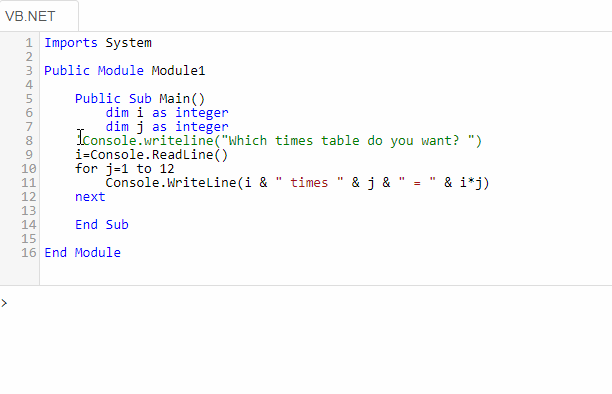The above example shows how a simple For Next loop can be used to produce times tables.

E.g.2 Coin Simulation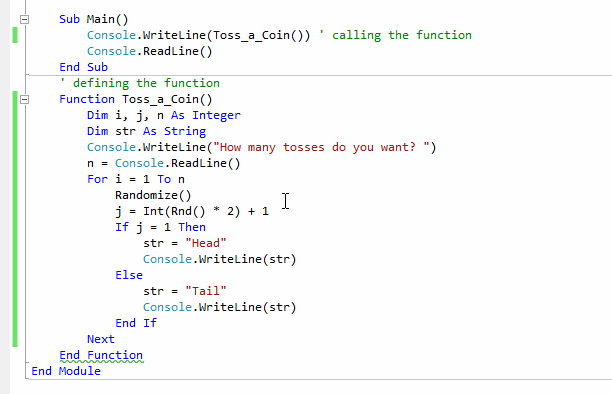The above example shows how a simple For Next loop can be used to simulate a coin.

Additive Persistence of a number is the number of steps needed for the sum of individual digits to be a single digit number, through multiple steps.

E.g. Let the number be 458
Step 1: 4 + 5 + 8 = 17
Step 2: 1 + 7 = 8
So, just in two steps, the sum of the individual digits becomes a single-digit number.

E.g. Let the number be 59
Step 1: 5 + 9 = 14
Step 2: 1 + 4 = 5
So, just in two steps, the sum of the individual digits becomes a single-digit number.

Now, let's use Visual Basic for the same purpose. Here is the code:

Sub Main()

Dim sum As Integer
Dim count As Integer
Dim number As Integer
Console.WriteLine("Enter a two-digit number between 11-99 ")
sum = Console.ReadLine() ' Get the input
number = sum 'Store the original number
count = 0
While sum > 9 'Making sure the sum has two digits
sum = (sum \ 10) + (sum Mod 10) ' Separating the two digits, before adding
count = count + 1 ' The counts goes up by 1 with each run
End While
Console.WriteLine("The Additive Persistence of " & number & " = " & count) 'Display the Additive Persistence

End Sub

Output:
Enter a two-digit number between 11-99
67
The Additive Persistence of 67 = 2

#### Converting Denary into Binary

This is a programme that converts any denary into binary form:

Sub Main()

Dim str As String = ""
Dim intemed As Integer
Console.WriteLine("Enter the denary number: ")
Dim nmbr As Integer = Console.ReadLine()
Dim number = nmbr
While number > 0
intemed = number Mod 2
str = CStr(intemed) + str
If number > 0 Then number = number \ 2
End While
Console.WriteLine("The binary value of " & nmbr & " = " & str)

End Sub

Output:
Enter the denary number:
33
The binary value of 33 = 100001

This is a programme that converts any denary into hexadecimal form:

Sub Main()

Dim str As String = ""
Dim intemed As Integer
Console.WriteLine("Enter the denary number: ")
Dim nmbr As Integer = Console.ReadLine()
Dim number = nmbr
While number > 0
intemed = number Mod 16
Select Case intemed
Case 0
str = CStr(0) + str
Case 1
str = CStr(1) + str
Case 2
str = CStr(2) + str
Case 3
str = CStr(3) + str
Case 4
str = CStr(4) + str
Case 5
str = CStr(5) + str
Case 6
str = CStr(6) + str
Case 7
str = CStr(7) + str
Case 8
str = CStr(8) + str
Case 9
str = CStr(9) + str
Case 10
str = "A" + str
Case 11
str = "B" + str
Case 12
str = "C" + str
Case 13
str = "D" + str
Case 14
str = "E" + str
Case Else
str = "F" + str
End Select
If number > 0 Then number = number \ 16
End While
Console.WriteLine("The hexadecimal value of " & nmbr & " = " & str)

End Sub

Output:
Enter the denary number:
2018
The hexadecimal value of 2018 = 7E2

#### Object Oriented Programming: OOP - Basic

A mathematician makes a simple app for kids in a certain primary school: it takes in the name, two numbers and the type of operation - +, -, x, :- - from a child and calculates what the child needs, while assigning the name of the child to the app.

The main focus here is the app - the Class
The nouns are the properties of the Class
The verbs are the methods - functions - of the Class

Since we identified the main parts of the class, let's build it as follows in the Console Module. The name of the class is Maths.

Module Module1

Public Class Maths

Public NumberOne As Integer
Public NumberTwo As Integer
Public Operation As String
Public Name As String

Public Sub New(YourName)
Me.Name = YourName
Console.WriteLine("This is an Object of class Maths; it belongs to: " & Name & ".")
End Sub

Public Function Calculate(first_number, second_number, what_to_do)
Me.NumberOne = first_number
Me.NumberTwo = second_number
Me.Operation = what_to_do
Select Case Operation
Return "The sum = " & first_number + second_number
Case "Subtract"
Return "The difference = " & first_number - second_number
Case "Multiply"
Return "The product = " & first_number * second_number
Case Else
Return "The quotation = " & first_number / second_number
End Select
End Function

End Class

End Module

The class diagram for the above class is as follows:

Maths
_____________________ NumberOne : Integer
NumberTwo : Integer
Operation : String
Name : String
_____________________ Calculate()

Based on this class, we can now make as many objects as we want - and use them. This is how four objects of the class Maths are formed and then used; please make sure they are created within Sub Main....End Sub.

Sub Main()

Dim App1 As Maths = New Maths("Addam")
Dim App2 As Maths = New Maths("Subtractra")
Console.WriteLine(App2.Calculate(12, 4, "Subtract"))
Dim App3 As Maths = New Maths("Producta")
Console.WriteLine(App3.Calculate(12, 4, "Multiply"))
Dim App4 As Maths = New Maths("Quotia")
Console.WriteLine(App4.Calculate(12, 4, "Divide"))

End Sub

Four objects are made from the above class: App1, App2, App3 and App4. The output of the above code is as follows:

This is an object of Class Maths. It belongs to Addam.
The sum = 16
This is an object of Class Maths. It belongs to Subtracta.
The difference = 8
This is an object of Class Maths. It belongs to Producta.
The product = 48
This is an object of Class Maths. It belongs to Quotia.
The quotient = 3

#### Constructor/s of the Class

Public Sub New(YourName) ...End Sub is called a constructor of the Class Maths. It's the first method to be initiated when the objects are created from the class.
The values that are to be assigned to the objects on creation, are usually placed inside a constructor. In this case, the name of the child is placed inside the constructor.
So, when object are created this must be provided as a parameter. Since the there is room for one variable in the constructor, it is called a parameterized constructor.
The constructor could have been used in the form of Public Sub New() ...End Sub too, without a parameter. Both constructors are allowed in the same class - with or without parameters; it depends on the need of the user.

You can make your own objects based on Class Maths and see the above code at work on the console on this page.

An advanced version of the OOP will be added to the tutorial soon...

The author of this page - and the site - is a Microsoft Certified Software Developer. The entire site is built on Microsoft .Net platform.Amazon Best SellerEverything is evolving; so is the layout of a text book. By uniquely presenting the rich contents of the book, the author has elevated positive user experience of reading a text book to a new level: the examples are easy to follow and rich in standard. Highly recommended for those who want to master JavaScript and JQuery.

### Progressive Web Apps(PWA)The significance of app stores is over; progressive web apps is the next big thing. They are just websites that makes the need of going through app stores and need of storing redundant. They work offline too. If you have a reasonable understanding of HTML, CSS and JavaScript, this is the book for you to learn in no time.

### HTML5 Canvas AnimationsDavid Geary, in this book, shows how to combine JavaScript and HTML5 Canvas to produce amazing animations; he has set aside a whole chapter to teach you the role of physics in animations. If you want an in-depth understanding about HTML5 Canvas animations, this is a must read.